# 一. 模式匹配

当有两个字符串`Str = "abdabcde;``modStr = "abcd";`时，如果要在Str中查找与modelStr相等的子串，则称Str为主串，modelStr为模式串。在查找过程中，从Str中的第一个字符进行比较，如果找到与modelStr相同的子串，函数返回modelStr字符串第一次出现的位置，否则返回-1。以上过程就称为模式匹配.

# 二. 模式匹配算法

模式匹配算法，最广为人知的有两种算法，一种为简单且暴力的BF算法，一种为效率极高的KMP算法。下文将会对两种方法进行详解。

## 1. 朴素模式匹配算法

BF算法为朴素模式匹配算法的经典，该算法的主要思想，从主串的第一个字符与模式串中的第一个字符进行比较，若相等则继续比较，若不相等则从主串中不相等的位置与模式串中的第一个字符进行比较。指针`i`指向主串，`j`指向模式串，当匹配失败时，`i`移动的位置为`i = i - j + 1`
当所有字符串匹配成功后，指针`j`指向了模式串末尾的下一个，在进行匹配的过程中，不难发现，当每次匹配失败后，`i`指针都进行了不必要的回溯，这个回溯过程造成了时间的大量浪费。

## 2. KMP算法

### 1). KMP算法的优势

上文讲述了BF算法的实现，也阐述了BF算法的效率低下之处，KMP算法就是针对BF算法的改良，当进行模式匹配时，出现字符比较不等的情况，不用回溯`i`指针，而是利用已经匹配过后的结果，向右尽可能滑动的稍远一些，再继续进行比较。

### 2). KMP算法的原理

以字符串`Str = "acabaabaabcacaabc";``modStr = "abaabcac";`为例，进行说明：
当`i=8, j=6`时，发现匹配失败: 如果按照BF算法， `i`指针会回溯至4的位置重新开始比较。但是这种`i`的回溯是有必要的吗？显然duck不必。
观察一下，`j1=j4, j2=j5且i3=j1,i4=j2`，所以回溯`i`指针到4的位置完全没有必要，根据上述等式关系，我们只需要将`j`指针的位置移动到`j4`的位置重新开始匹配。再看一个例子: `str=“aabababcaad” modStr=“babc”`，当`i=6, j=4`时发生失配，但`j1=j3`,所以直接将`j`指针移动到`j4`的位置，开始匹配。再看最后一个例子，`str=“aacabcd”, modStr=“abcd`,当发生失配时，怎么操作。
在发生失配时，将i指针向后移动一位，继续进行比较。综合上述例子，不难看出模式串中存在着字符相同的元素，在模式串的子串中构成了前缀与后缀。并且在失配之后对于`i指针的移动存在一定的规律，从而引出了next数组的概念，next数组用于存放模式匹配失配时，模式串的滑动距离。

### 3). next数组的构造

通过上述的例子，已经得知`next[]`数组存放的内容为，模式串与目标串匹配失败时用于寻找模式串回溯位置的数组。
如何计算next数组呢？其中最重要的概念就是最大前缀与最大后缀。公式是这样表示最大前缀与最大后缀的，但是实际中如何求解呢。`str="abaabcac"`以这个字符串为例，表示出它的前缀与后缀。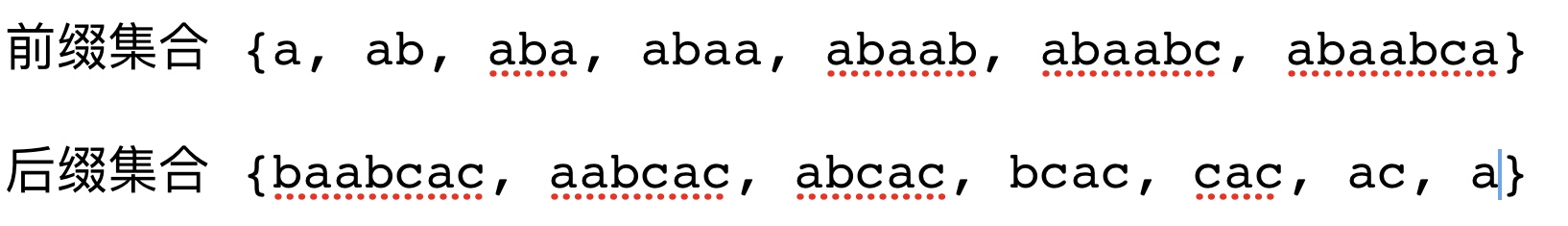对于这个字符串它的前缀与后缀是这样的，所以它的最大前缀=最大后缀的字符串是`a`;
接下来就要通过这个最大前缀=最大后缀的概念，来进行失配后模式串回溯的位置计算。

设模式串为`abaabcac`( `j`指向的位置为当前匹配位置，假设在当前位置失配，求出在当前位置失配的回溯位置，`j`指针前方字符串为要计算回溯位置的子串)
1.j=1失配 (因为j=0 所以next[j]=0)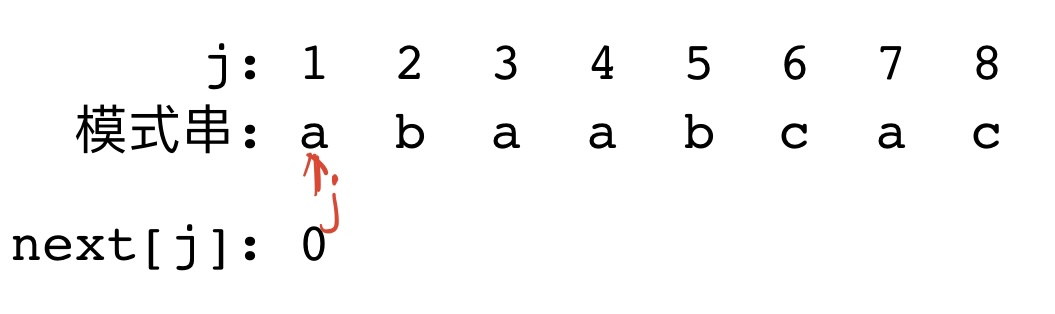2.j=2失配 (没有前后缀，属于其他情况)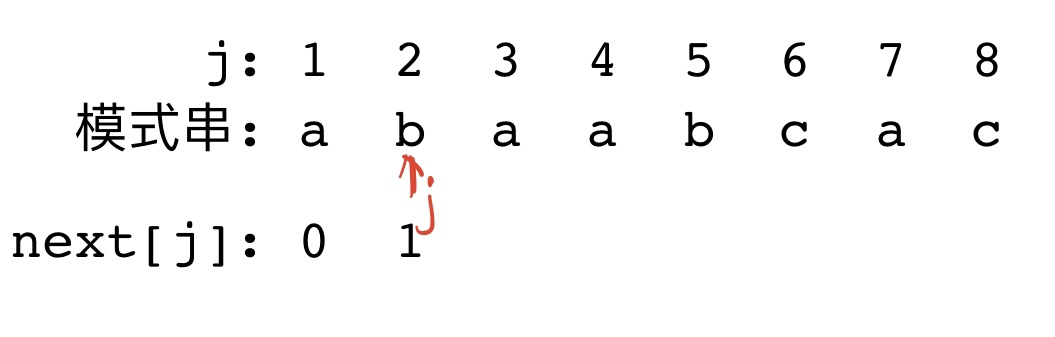3.j=3失配(前缀不等与后缀)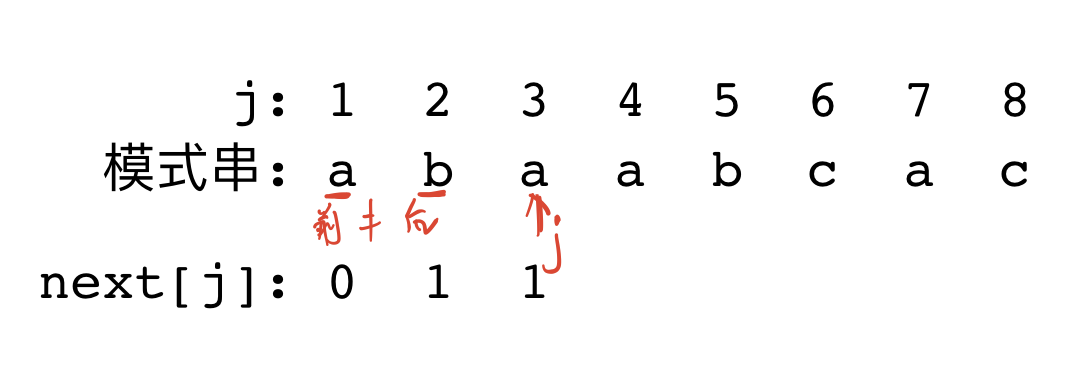4.j=4失配(最大前缀=最大后缀,所以k=2)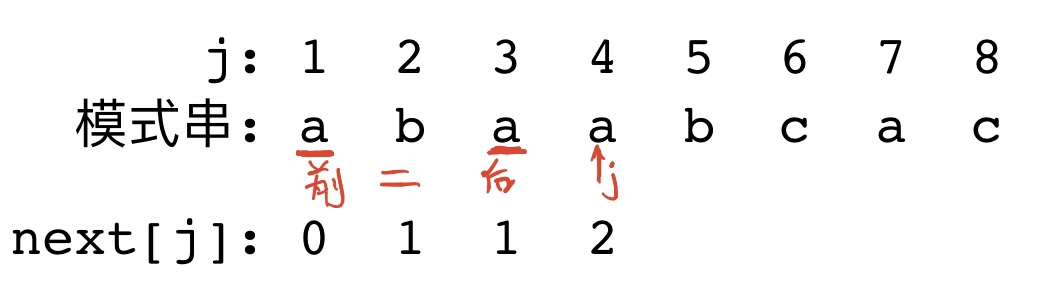5.j=5失配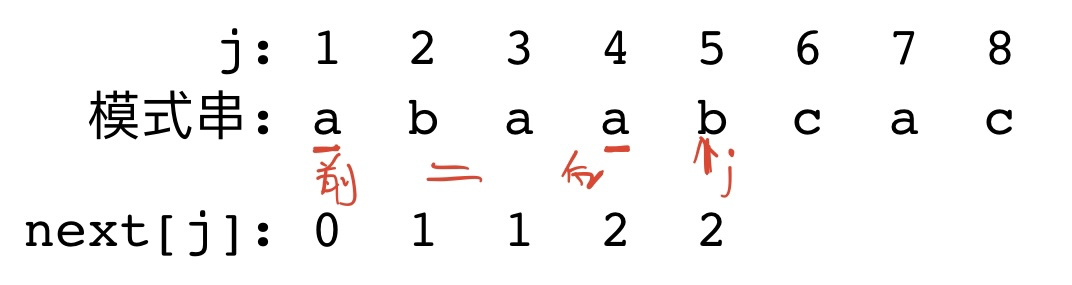6.j=6失配 (最大前缀 ab = 最大后缀 ab, 所以k=3)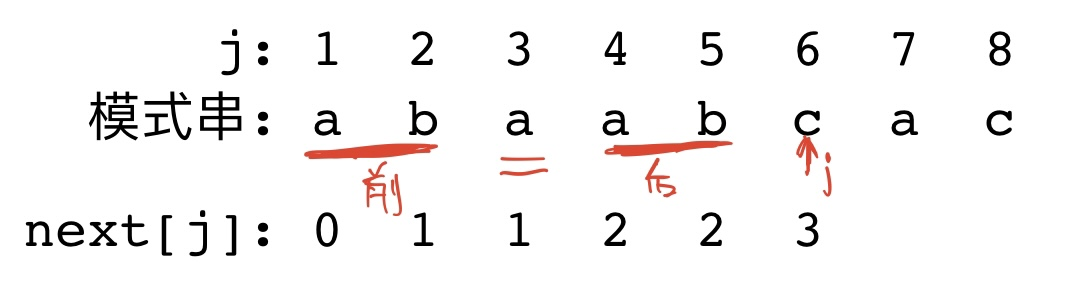7.j=7失配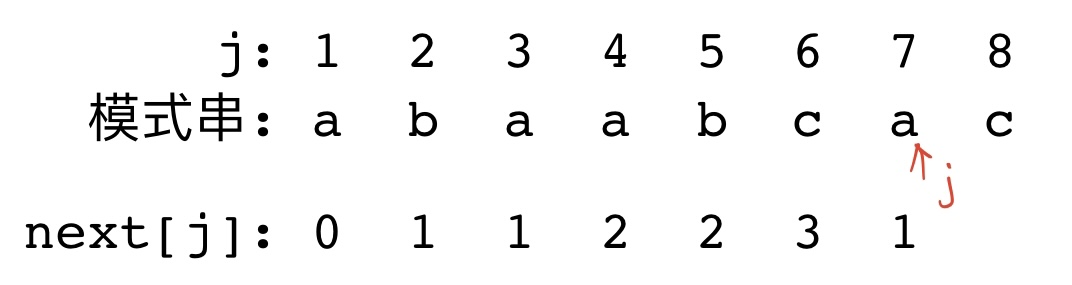8.j=8失配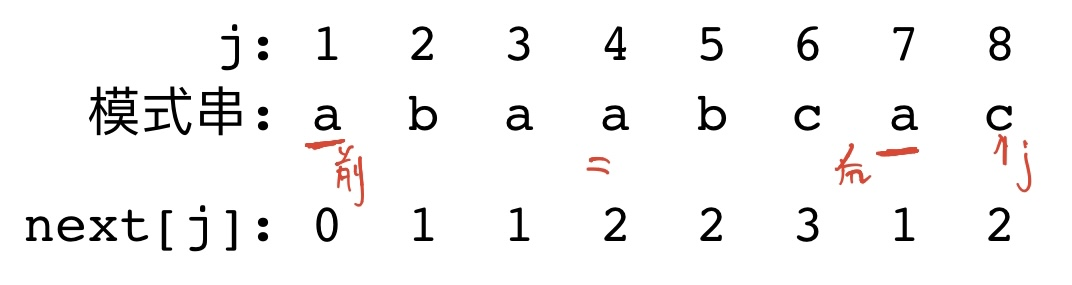以上为next数组求法，但字符串下表一般从0开始，所以要对next数组中元素-1，得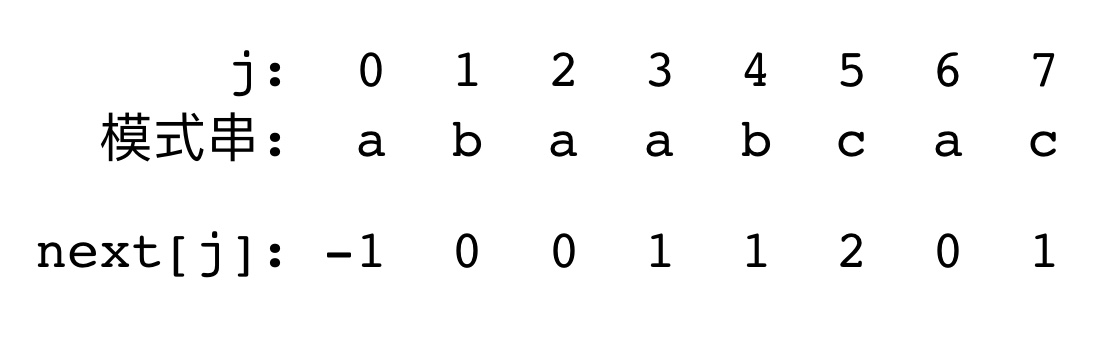### 4). 利用next数组匹配的过程

在这一部分，将会说明模式匹配是如何利用next数组进行模式匹配。
以`str = "acabaabaabcacaabc";``modStr = "abaabcac";`为例，字符串下标从0开始，进行说明：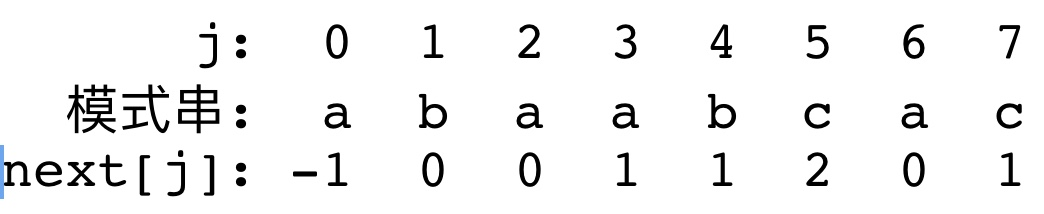1.第一次匹配(i=1,j=1位置发生失配)

2.第二次匹配(i=1,j=0位置发生失配)
next数组中没有相关跳转位置，所以`i`指针后移一位，开始匹配。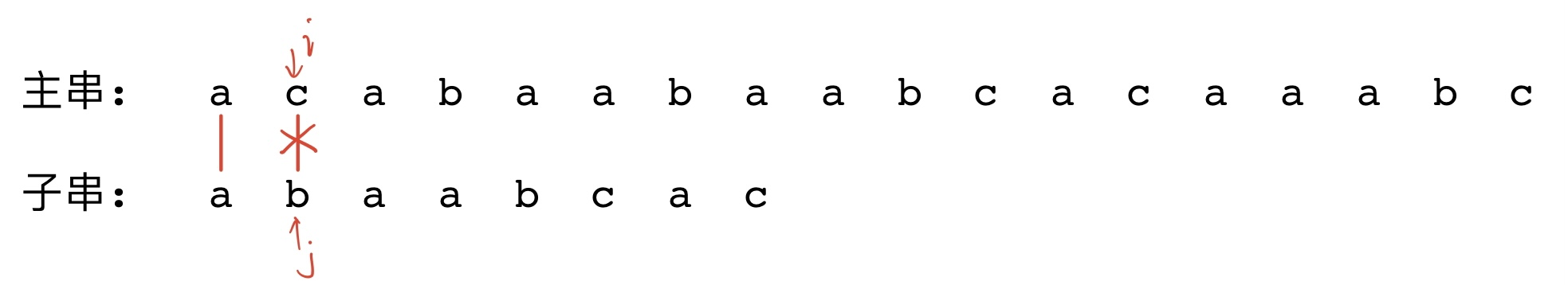3.第三次匹配(i=7,j=5位置发生失配)

4.匹配成功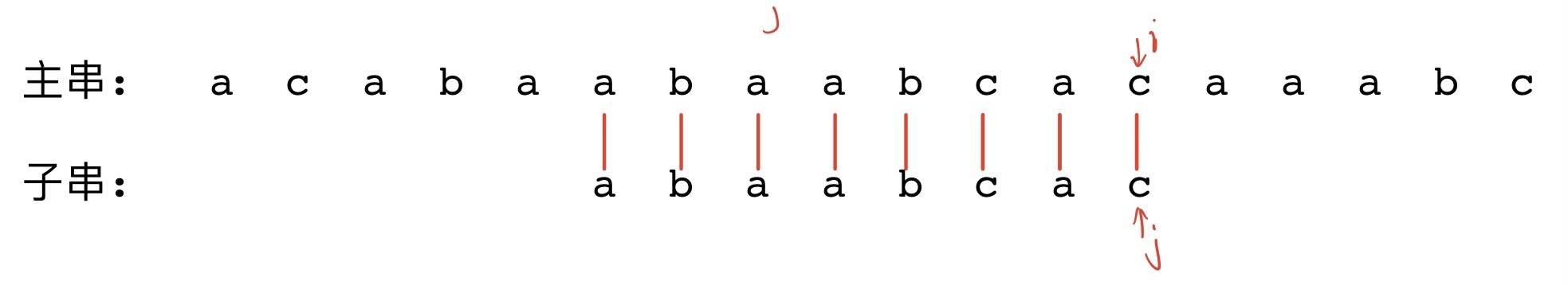# 二. KMP算法的代码实现

## 1. 生成next[]数组

``````void getNextArr(string modelStr, int* next)
{
// 初始化next数组第一位为-1
int i = 0;
int j = -1;
next = -1;

int mLen = modelStr.length();

while (i < mLen - 1)
{
// 求最大前缀=最大后缀的过程
if (j == -1 || modelStr[i] == modelStr[j])
{
i++;
j++;
next[i] = j;
}
else
{
// 当没有匹配上 则进行回溯 回溯的位置为next数组的指向的下一个匹配项
j = next[j];
}
}
}
``````

## 2. KMP查找过程代码

``````// 如果查找成功返回位置
// 如果查找失败返回-1
int KMP(string dstStr, string modelStr)
{
int i = 0;
int j = 0;

int dstlen = dstStr.length();
int modellen = modelStr.length();

int next = { 0 };

getNextArr(modelStr, next);

while ((i < dstlen) && (j < modellen))
{
if (j == -1 || dstStr[i] == modelStr[j])
{
i++;
j++;
}
else
{
j = next[j];
}
}

if (j >= modellen)
{
return i - modellen;   // 匹配成功 返回子串位置
}
else
{
return -1;
}
}
``````